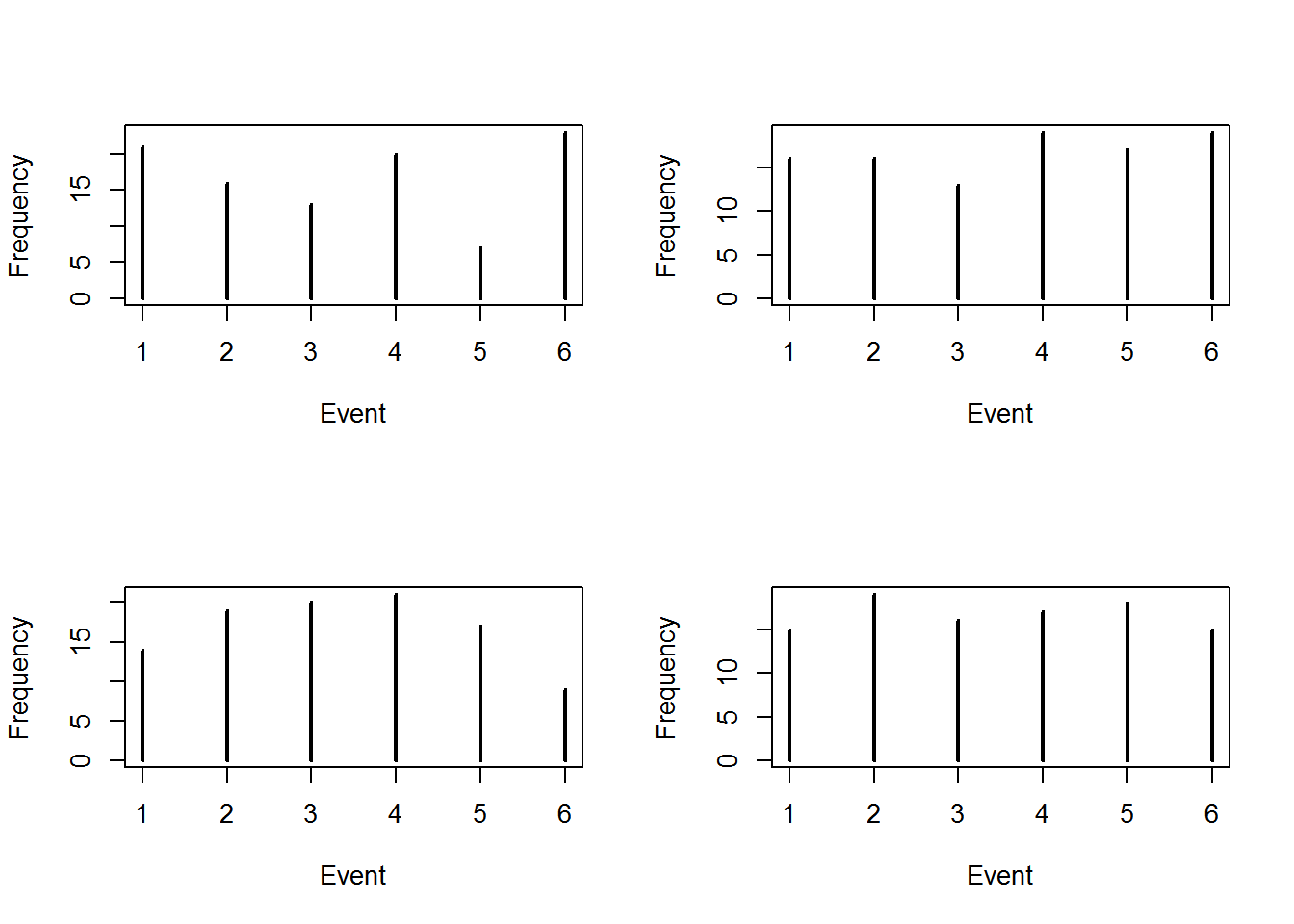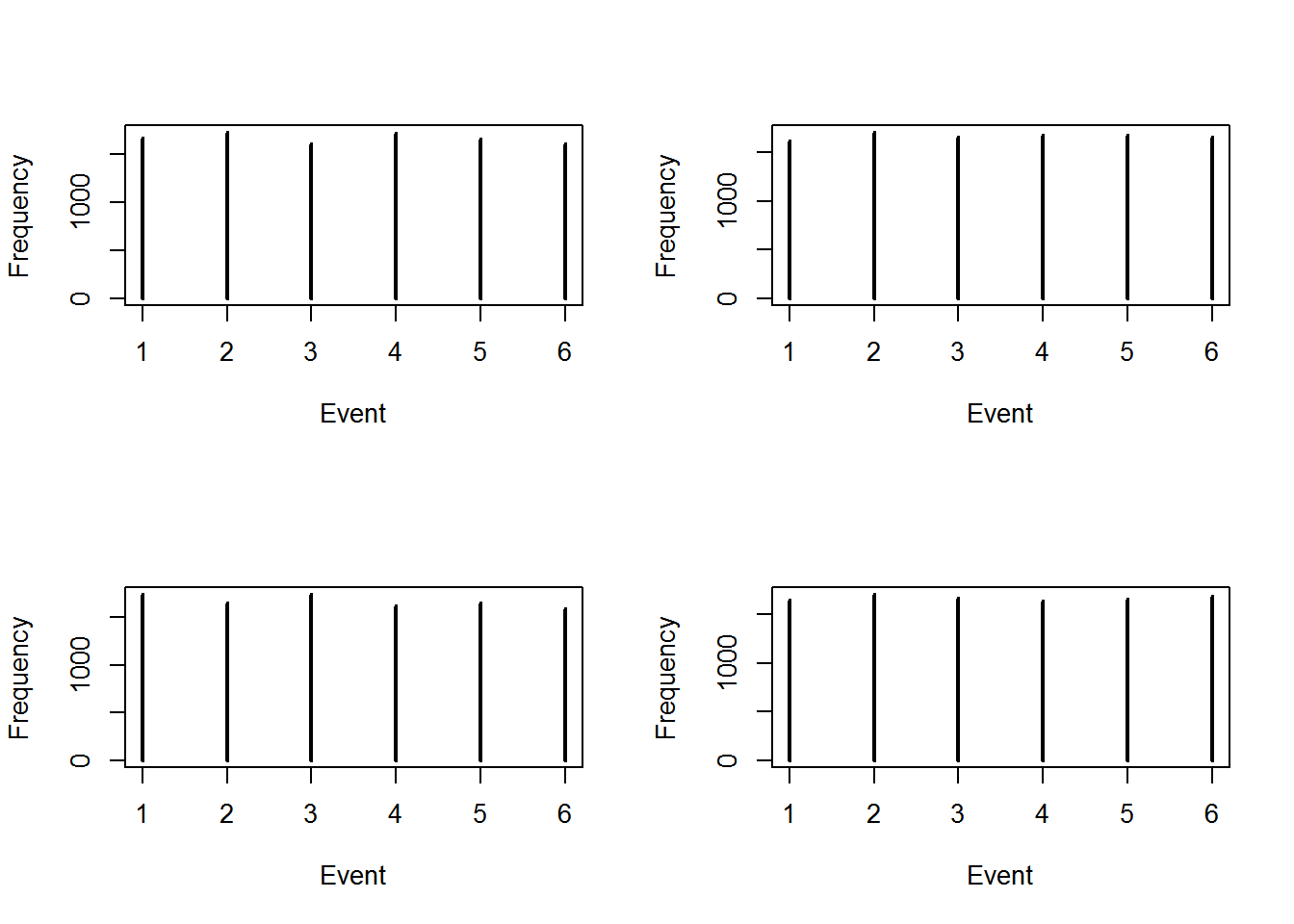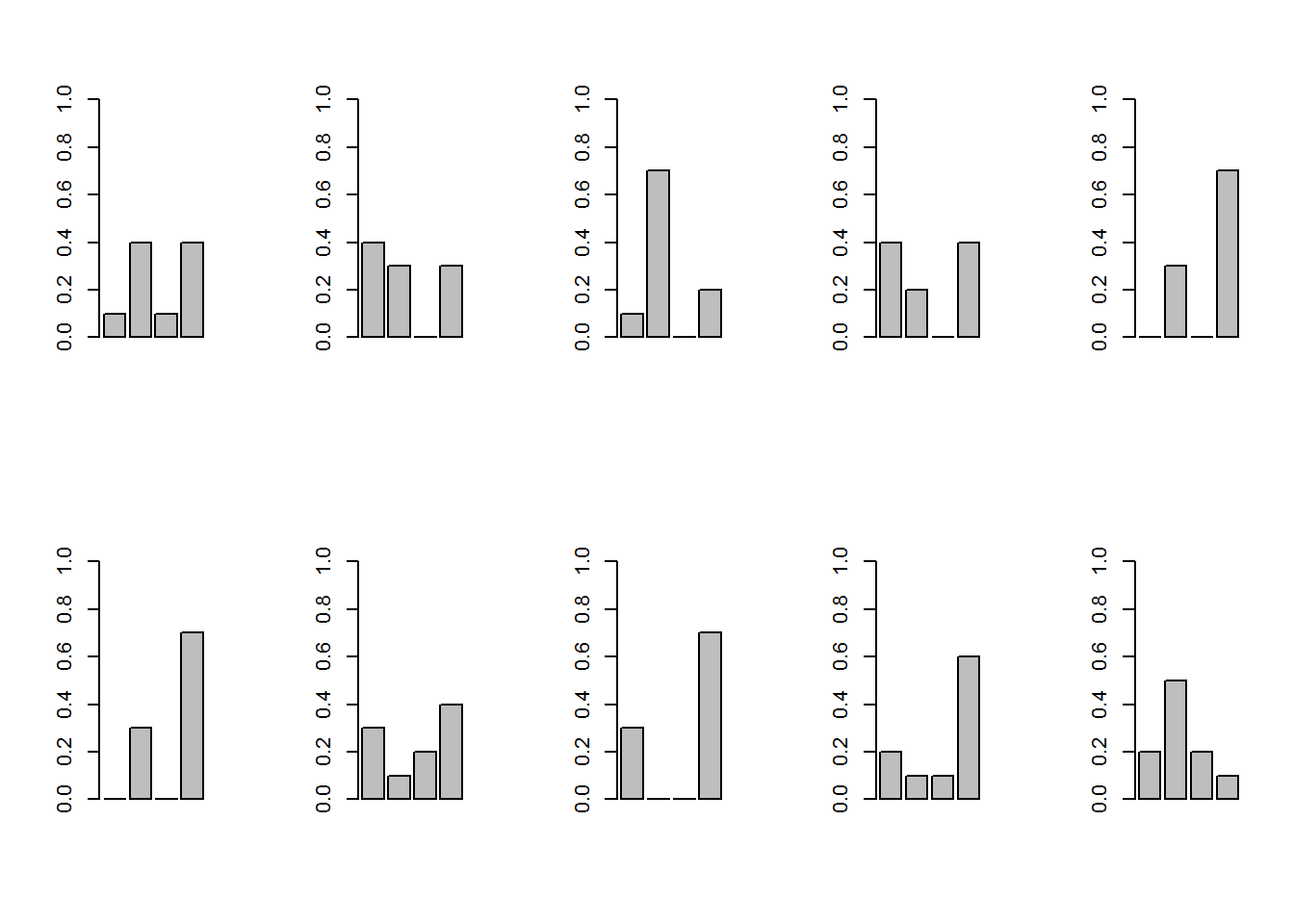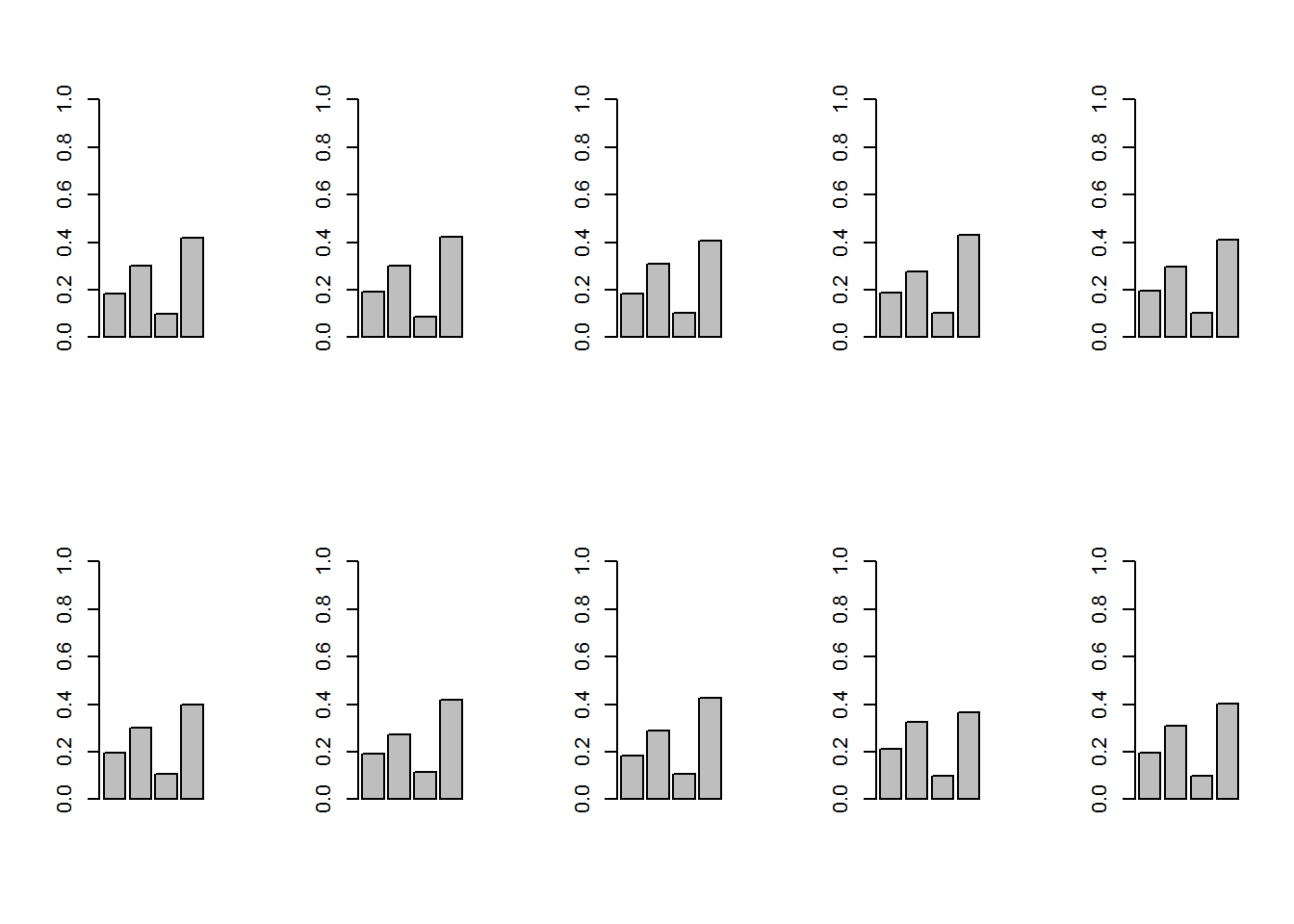## Multinomial Distribution Basics

The multinomial distribution is a generalization of the binomial distribution to k categories instead of just binary (success/fail). For n independent trials each of which leads to a success for exactly one of k categories, the multinomial distribution gives the probability of any particular combination of numbers of successes for the various categories

Example: Rolling a die N times

In Data mining, When we discuss everything in terms of text classification, i.e. Topic Modeling:

Each document has its own distribution over topics. Each topic has its own distribution over the words.

The (multinomial) distribution over words for a particular topic The (multinomial) distribution over topics for a particular document

## Chess Game Prediction

Two chess players have the probability Player A would win is 0.40, Player B would win is 0.35, game would end in a draw is 0.25.

The multinomial distribution can be used to answer questions such as: “If these two chess players played 12 games, what is the probability that Player A would win 7 games, Player B would win 2 games, the remaining 3 games would be drawn?”

dmultinom(x=c(7,2,3), prob = c(0.4,0.35,0.25))
##  0.02483712

## Opinion Polls on Election

In a little town, 40% of the eligible voters prefer candidate A, 10% prefer candidate B, 50% have no preference.

You randomly sample 10 eligible voters. What is the probability that 4 will prefer candidate A, 1 will prefer candidate B, 5 will have no preference ?

dmultinom(x=c(4,1,5), prob = c(0.4,0.1,0.5))
##  0.1008

## Throwing Dice as Multinomial Distribution

A distribution that shows the likelihood of the possible results of a experiment with repeated trials in which each trial can result in a specified number of outcomes that is greater than two. A multinomial distribution could show the results of tossing a dice, because a dice can land on one of six possible values. By contrast, the results of a coin toss would be shown using a binomial distribution because there are only two possible results of each toss, heads or tails.

Two additional key characteristics of a multinomial distribution are that the trials it illustrates must be independent (e.g., in the dice experiment, rolling a five does not have any impact on the number that will be rolled next) and the probability of each possible result must be constant (e.g., on each roll, there is a one in six chance of any number on the die coming up).

### Rolling a die N=100 times

one.dice <- function(){
dice <- sample(1:6, size = 1, replace = TRUE)
return(dice)
}

one.dice()
##  4
one.dice()
##  2
one.dice()
##  3
par(mfrow=c(2,2))

for (i in 1:4){
sims <- replicate(100, one.dice())
table(sims)
table(sims)/length(sims)
plot(table(sims), xlab = 'Event', ylab = 'Frequency')
}### Rolling a die N=10000 times

par(mfrow=c(2,2))

for (i in 1:4){
sims <- replicate(10000, one.dice())
table(sims)
table(sims)/length(sims)
plot(table(sims), xlab = 'Event', ylab = 'Frequency')
}## Generate Multinomial Random Variables

Generate Multinomial Random Variables With Varying Probabilities Given a matrix of multinomial probabilities where rows correspond to observations and columns to categories (and each row sums to 1), generates a matrix with the same number of rows as has probs and with m columns. The columns represent multinomial cell numbers, and within a row the columns are all samples from the same multinomial distribution.

# rmultinom(n, size, prob)
my_prob <- c(0.2,0.3,0.1,0.4)
number_of_experiments <- 10

### Number of Samples = 10

number_of_samples <- 10

experiments <- rmultinom(n=number_of_experiments, size=number_of_samples, prob=my_prob)
experiments
##      [,1] [,2] [,3] [,4] [,5] [,6] [,7] [,8] [,9] [,10]
## [1,]    1    4    1    4    0    0    3    3    2     2
## [2,]    4    3    7    2    3    3    1    0    1     5
## [3,]    1    0    0    0    0    0    2    0    1     2
## [4,]    4    3    2    4    7    7    4    7    6     1
df=data.frame(experiments)/number_of_samples

par(mfrow=c(2,5))

for(i in 1:10) {
barplot(df[,i],ylim=c(0,1))
}### Number of Samples = 1000

number_of_samples <- 1000

experiments <- rmultinom(n=number_of_experiments, size=number_of_samples, prob=my_prob)
experiments
##      [,1] [,2] [,3] [,4] [,5] [,6] [,7] [,8] [,9] [,10]
## [1,]  184  193  182  188  194  196  193  182  211   194
## [2,]  301  300  309  278  296  302  274  288  327   307
## [3,]   98   86  103  103  101  105  113  105   97    98
## [4,]  417  421  406  431  409  397  420  425  365   401
df=data.frame(experiments)/number_of_samples

par(mfrow=c(2,5))

for(i in 1:10) {
barplot(df[,i],ylim=c(0,1))
}